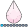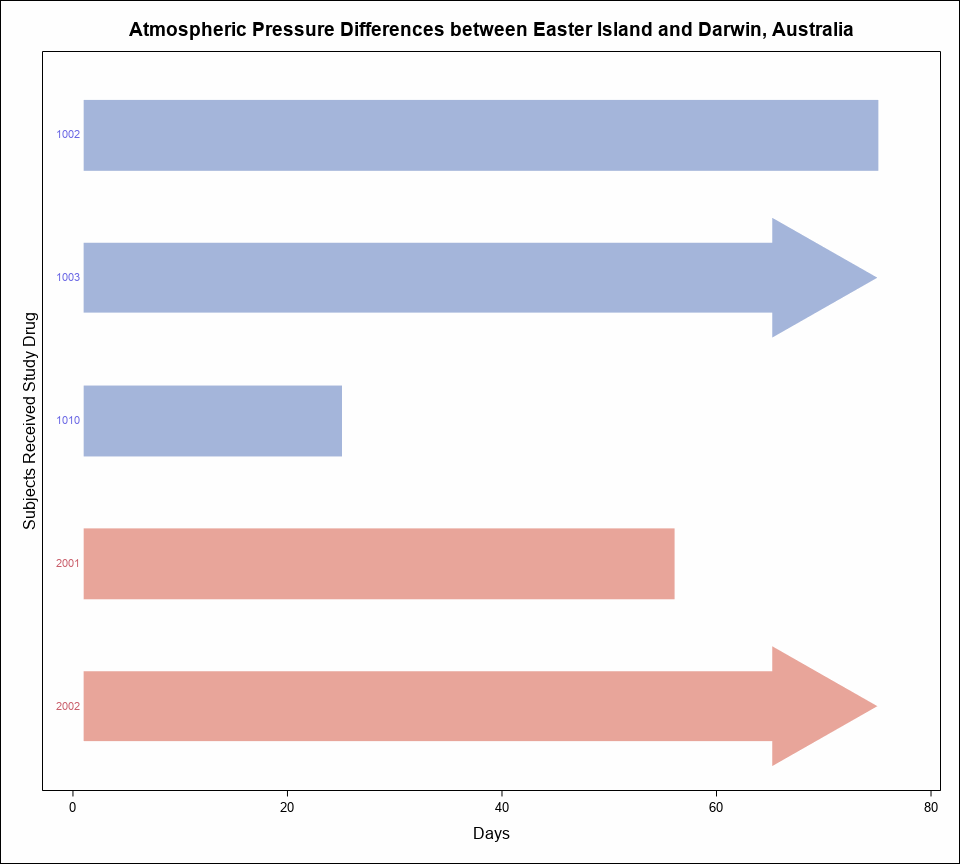## Proc SGPLO HIGHCAP option not working

I am trying to plot a high low bar in SAS using proc sgplot procedure. The sample data and code is below. No matter I do, I do not see the FilledArrow when the end day is missing. In fact sas is not even plotting these subjects. When Start and End both are present it is working fine but not when start is present but end is missing. In that case these subjects are not plotted and I do not see FilledArrow.  Any help is appreciated.

Sample Data

Subject   Start   End    ACAP              Project

1010        1         25                                A

1002        1        75                                 A

1003        1        .         FilledArrow         A

2001        1        56                                  B

2002         1       .         FilledArrow           B

...

proc sgplot data=final noautolegend nocycleattrs;

highlow y=subject low=start high=end / highcap=acap group=project type=bar nooutline nomissinggroup lowlabel = subject transparency=0.3 ;

xaxis label='Days' valueshint;

yaxis reverse display=(noticks novalues noline) label='Subjects Received Study Drug' min=1;

run;

1 ACCEPTED SOLUTION

Accepted Solutionsdjrisks
Barite | Level 11

## Re: Proc SGPLO HIGHCAP option not working

Hello Leo,

I believe that you should still impute end values for subject 1003 and 2002. You could impute the value of 75, or choose a smaller or larger value, depending on how you want to represent missing values.

``````data final2;
set final;
if end = . then end = 75;
run;

proc sgplot data=final2 noautolegend nocycleattrs;
highlow y=subject low=start high=end / highcap=acap group=project type=bar nooutline nomissinggroup lowlabel = subject transparency=0.3 ;
xaxis label='Days' valueshint;
yaxis type = discrete reverse display=(noticks novalues noline) label='Subjects Received Study Drug' min=1;
run;``````djrisks
Barite | Level 11

## Re: Proc SGPLO HIGHCAP option not working

Hello Leo,

I believe that you should still impute end values for subject 1003 and 2002. You could impute the value of 75, or choose a smaller or larger value, depending on how you want to represent missing values.

``````data final2;
set final;
if end = . then end = 75;
run;

proc sgplot data=final2 noautolegend nocycleattrs;
highlow y=subject low=start high=end / highcap=acap group=project type=bar nooutline nomissinggroup lowlabel = subject transparency=0.3 ;
xaxis label='Days' valueshint;
yaxis type = discrete reverse display=(noticks novalues noline) label='Subjects Received Study Drug' min=1;
run;``````Discussion stats